Function Repository Resource:

# KirchhoffHypergraph

Compute the hypergraph with a specified Kirchhoff tensor (Laplacian)

Contributed by: Jonathan Gorard
 ResourceFunction["KirchhoffHypergraph"][ktens] gives the (ordered or orderless) hypergraph with Kirchhoff tensor ktens.

## Details and Options

A Kirchhoff tensor (hypergraph Laplacian) is a generalization of the concept of a Kirchhoff matrix (graph Laplacian) from graphs to hypergraphs, in which hyperedges may be of arbitrary arity.
The arity of the hyperedges in the hypergraph is equal to the rank of the Kirchhoff tensor.
The number of vertices will be equal to n, where the dimensions of the adjacency tensor are n×n××n.
ResourceFunction["KirchhoffHypergraph"] accepts Kirchhoff tensors specified both as SparseArray objects, and as ordinary nested lists.
The degree of the vertex vi is given by the diagonal entry aii…i of the Kirchhoff tensor.
The number of hyperedges of the form {vi,vj,,vk} is specified by the off-diagonal entry -aij…k of the Kirchhoff tensor.
ResourceFunction["KirchhoffHypergraph"] has the following option:
 "OrderedHyperedges" Automatic whether to treat hyperedges as being ordered (directed)
The following settings for "OrderedHyperedges" can be used in ResourceFunction["KirchhoffHypergraph"]:
 Automatic construct an orderless hypergraph if and only if the Kirchhoff tensor is symmetric across all indices True construct an ordered hypergraph False construct an orderless hypergraph

When the Kirchhoff tensor is of rank 2, the output of ResourceFunction["KirchhoffHypergraph"] is identical to that of KirchhoffGraph.

## Examples

### Basic Examples (3)

Construct an ordered hypergraph automatically from an asymmetric Kirchhoff tensor:

 In:=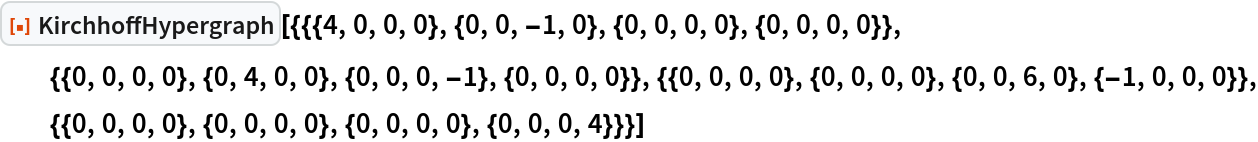Out=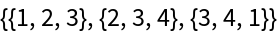Construct an orderless hypergraph automatically from a symmetric Kirchhoff tensor:

 In:=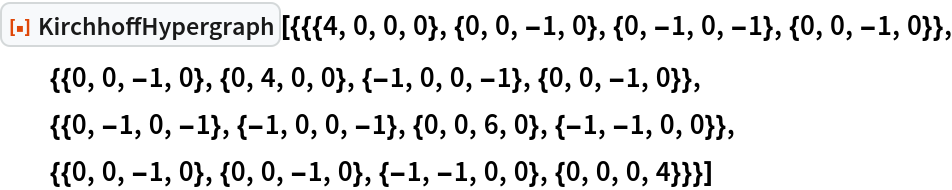Out=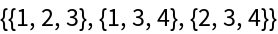Treat the hypergraph as being ordered instead:

 In:=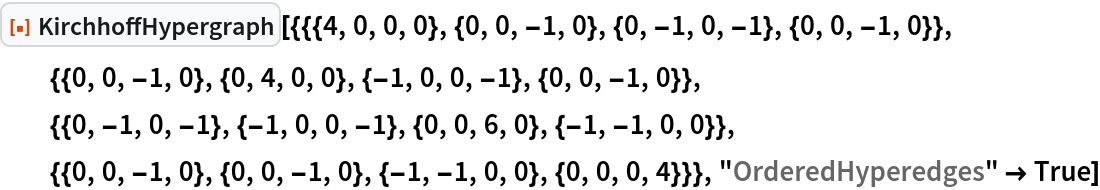Out=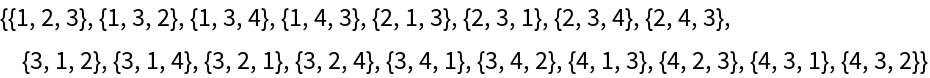Construct an ordered hypergraph of arity 5 automatically from an asymmetric Kirchhoff tensor specified as a SparseArray:

 In:=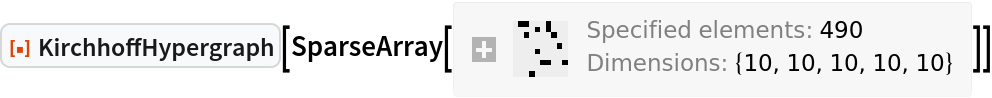Out=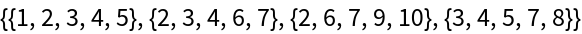### Scope (6)

KirchhoffHypergraph accepts both SparseArray and nested list specifications of Kirchhoff tensors:

 In:=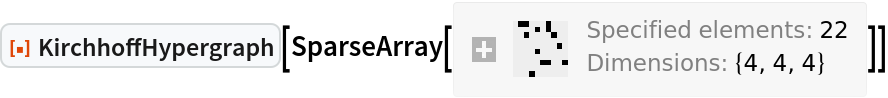Out=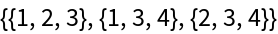In:=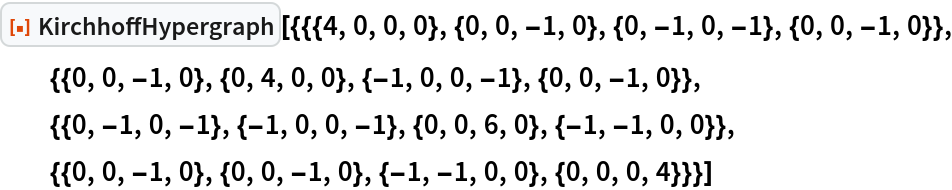Out=KirchhoffHypergraph supports multihypergraphs, with off-diagonal Kirchhoff tensor entries representing hyperedge multiplicities:

 In:=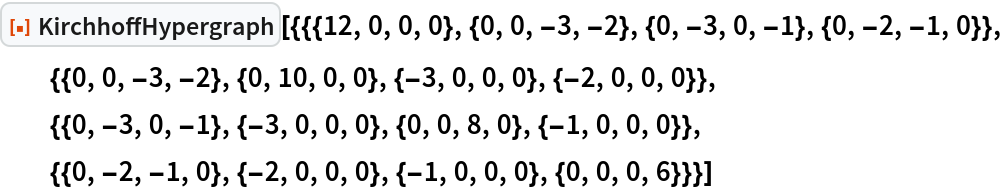Out=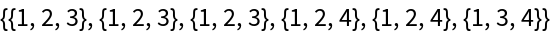When the rank of the Kirchhoff tensor is equal to 2, the output of KirchhoffHypergraph is identical to the output of KirchhoffGraph:

 In:=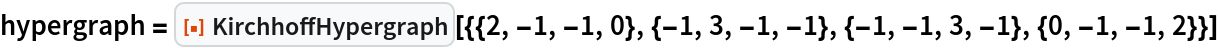Out=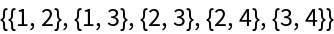In:=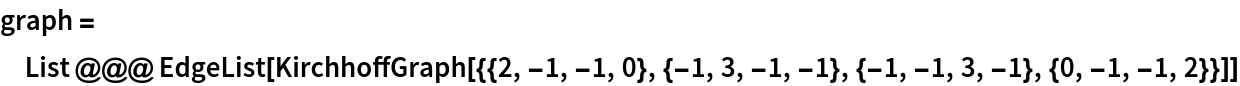Out=In:=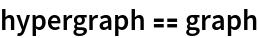Out=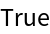When the Kirchhoff tensor is symmetric across all indices, the hypergraph is automatically orderless:

 In:=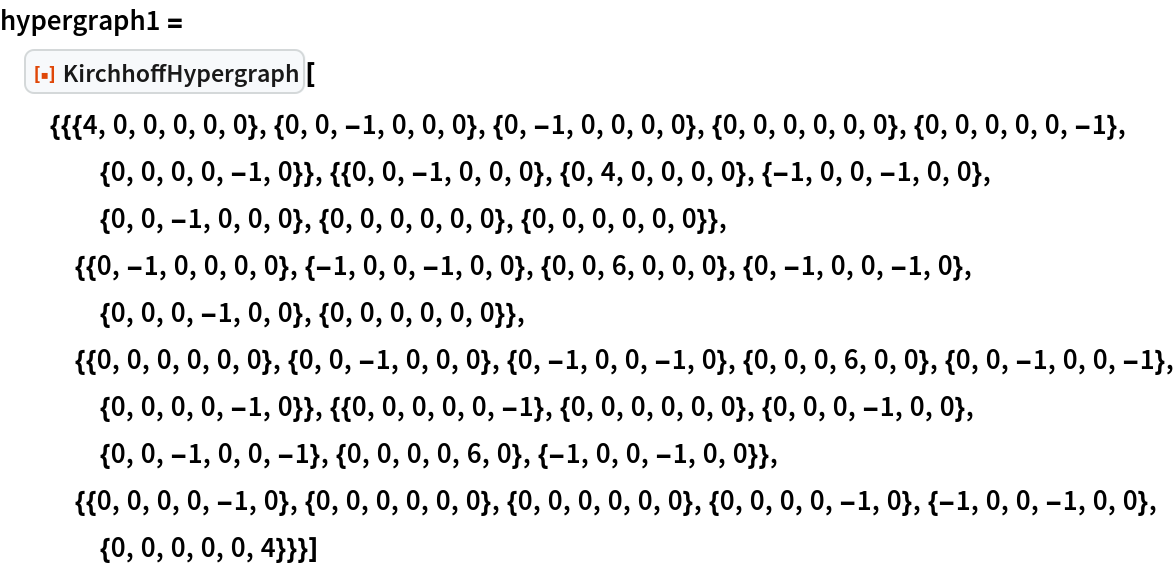Out=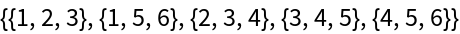In:=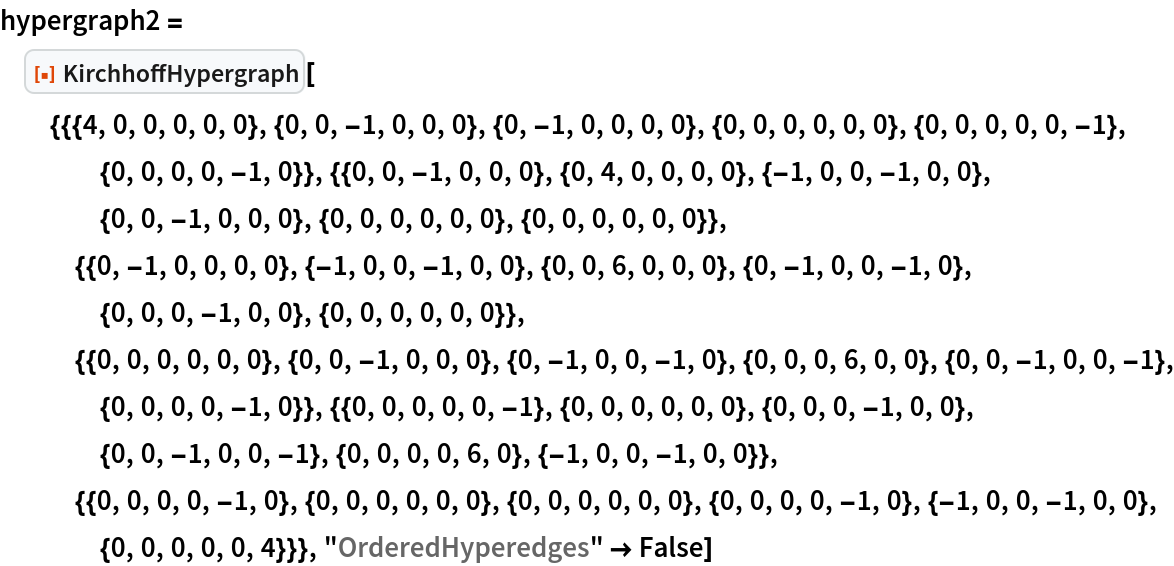Out=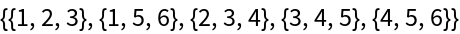In:=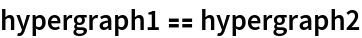Out=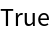When the Kirchhoff tensor is asymmetric across any pair of indices, the hypergraph is automatically ordered:

 In:=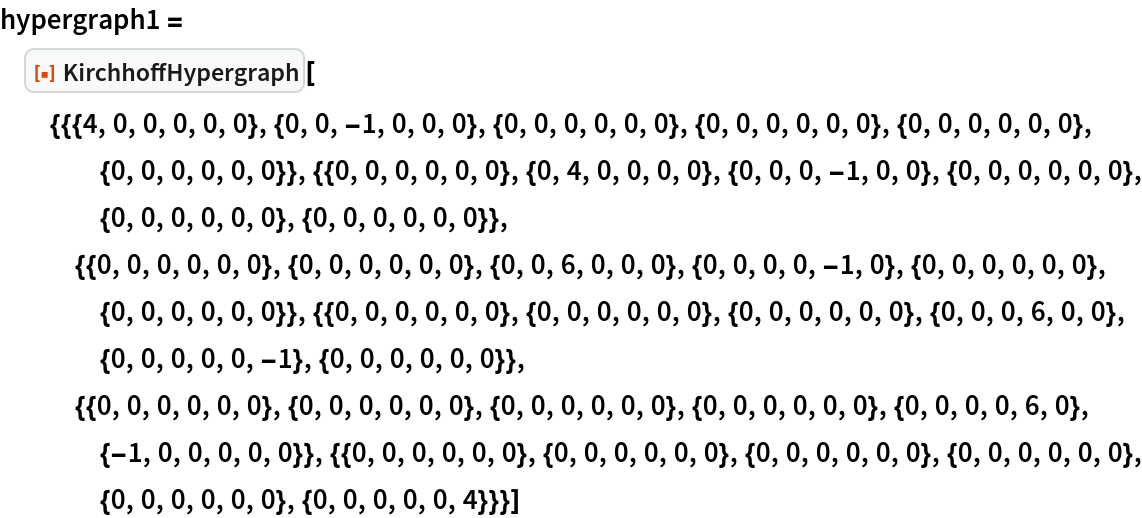Out=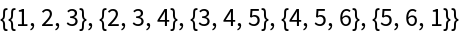In:=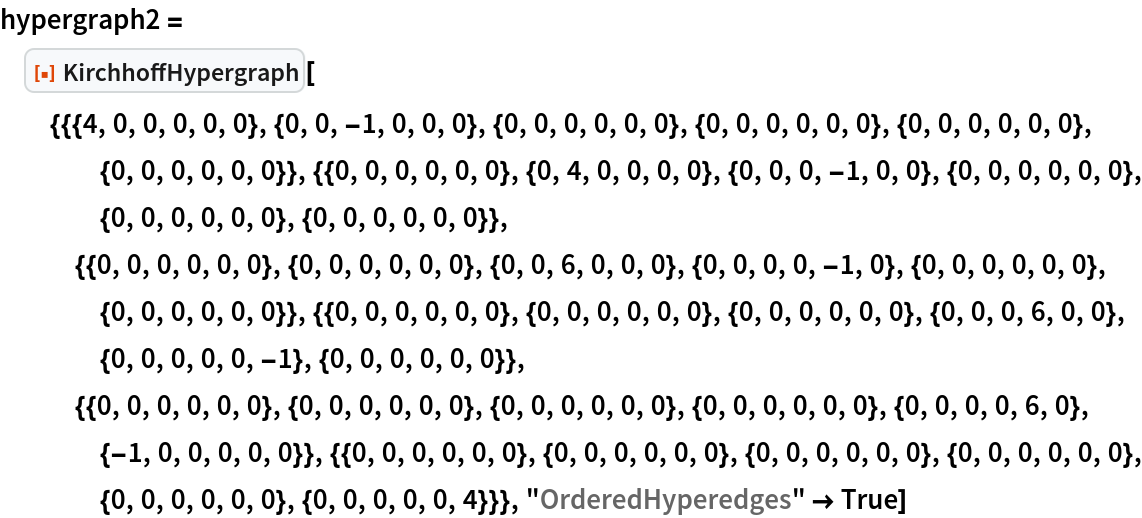Out=In:=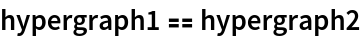Out=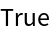Kirchhoff tensors can be of arbitrary rank:

 In:=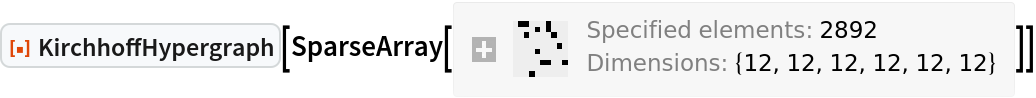Out=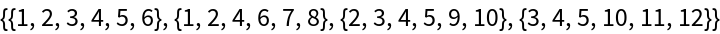### Options (3)

By default ("OrderedHyperedges"Automatic), all hyperedges are treated as orderless (i.e. undirected) if the Kirchhoff tensor is symmetric across all indices:

 In:=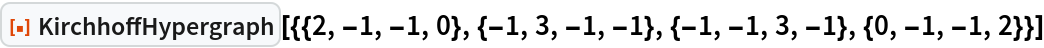Out=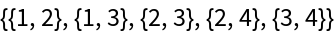Use "OrderedHyperedges"True to treat hyperedges as ordered (i.e. directed):

 In:=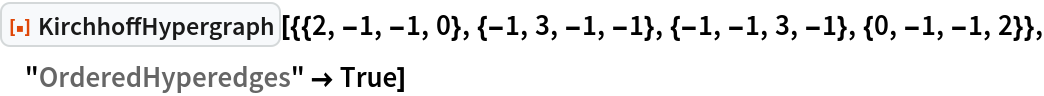Out=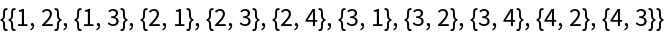Conversely, all hyperedges are treated as ordered (i.e. directed) if the Kirchhoff tensor is asymmetric across any pair of indices:

 In:=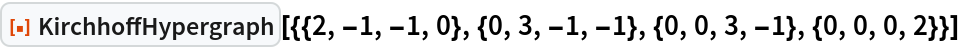Out=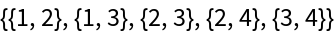Jonathan Gorard

## Version History

• 1.0.0 – 17 March 2021